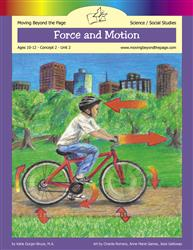# Force and Motion Age 10-12: Concept 2 - Force and Power: Unit 2Learn about different types of forces and how they affect motion through hands-on experiments involving gravity, magnetism, buoyancy, and more. Explore Newton's laws of motion and demonstrate how simple machines make work easier.

This unit can be used independently, but it is designed to be used in conjunction with the language arts unit Albert Einstein.
#1083
\$39.94
#1084
\$32.88
#2476
\$4.99

## Prerequisites

• Able to write multiple paragraphs on a topic
• Familiar with the five-paragraph essay
• Usually used by children in the sixth grade

• Lesson 1: Force and Motion Basics
• Lesson 2: Forces (2 Days)
• Lesson 3: Gravity (3 Days)
• Lesson 4: Laws of Motion (2 Days)
• Lesson 5: Magnetism
• Lesson 6: Buoyancy
• Lesson 7: Forces at Work (2 Days)
• Final Project: Force and Motion Stations (3 Days)

## Summary of Skills

Moving Beyond the Page is based on state and national standards. These standards are covered in this unit.
• Build and use a model to solve a mechanical design problem, including testing and evaluating the results of the test. (Science)
• Calculate average speed using distance and time measurements. (Science)
• Compare and contrast potential and kinetic energy. (Science)
• Complete demonstrations that test the effect of forces on an object. (Science)
• Conduct an investigation to provide evidence that fields exist between objects exerting forces on each other even though the objects are not in contact. (Science)
• Demonstrate the effect of simple machines (lever, inclined plane, pulley, wedge, screw, and wheel and axle) on work. (Science)
• Design an experiment that tests the effect of force on an object. (Science)
• Design and conduct investigations to demonstrate an understanding of scientific inquiry. (Science)
• Determine factors that affect motion, including force. (Science)
• Determine how friction affects motion. (Science)
• Determine how inertia affects motion. (Science)
• Determine how momentum affects motion. (Science)
• Determine how people use simple machines to solve problems. (Science)
• Determine the motion of an object by following and measuring its position over time. (Science)
• Evaluate how pushing or pulling forces can change the position and motion of an object. (Science)
• Evaluate how the force of gravity affects objects. (Science)
• Explain how energy is needed to make machines move against the force of gravity. (Science)
• Explain how energy is needed to make machines move against the force of moving air. (Science)
• Explore how inertia and momentum affect motion. (Science)
• Identify and describe the changes in direction, motion, and speed of an object when acted upon by unbalanced forces. (Science)
• Identify and explain how forces act on objects. (Science)
• Identify and explain how magnetism acts on objects. (Science)
• Identify simultaneous force pairs that are equal in magnitude and opposite in direction. (Science)
• Investigate the relationship between force and motion using a variety of means. (Science)
• Model how air resistance affects gravitational force. (Science)
• Understand the basic vocabulary related to force and motion. (Science)
← go back